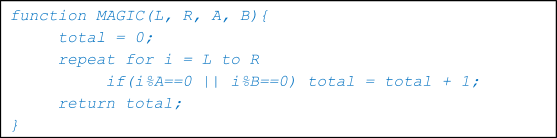Problems
10148 - Pseudo-Queen

Pseudo-Queen

Time Limit: 1 sec

The Problem

“ Hey pseudo-queen. ”

I call my friend Tithi by this name. She is a nice pseudo code solver. Whenever she got a pseudo code she tries to crack the code into smaller parts and figure out what’s going on with each and every part. But today she seems to be very anxious with a pseudo code. I never seen her so much anxious before as today she is. She gives me that pseudo code and she also told that she will call me as “Pseudo-King” if I help her. The pseudo code is given below -What you have to do with this pseudo code is – “You are given L, R, A, B. You need to find the result of MAGIC(L, R, A, B).

As you know I am a very bad student so I give you the same pseudo code to you. Please help me in getting the “Pseudo-King” title by solving this. And don’t tell her that you helped me.  :-P

The Input

Input starts with an integer T which denotes the number of test cases you need to perform.  Each of the next T lines you will be given 4 integers L, R, A, B.

Constraints

1<= T <= 10^5

1<= ( L <= R ), A, B <= 2^63 – 1

The Output

For each test case print the case number and the result of MAGIC(L, R, A, B) as the following format “Case X: Y” where X is the case number starts from 1 and Y is the desired answer.  Check the samples for further clarification.

Sample Input

2

1 10 2 3

3 5 2 3

Sample Output

Case 1: 7

Case 2: 2

Inter University Programming Contest(I) - 2018 [BGC Trust University Bangladesh]

Problem Setter: Avik Sarkar